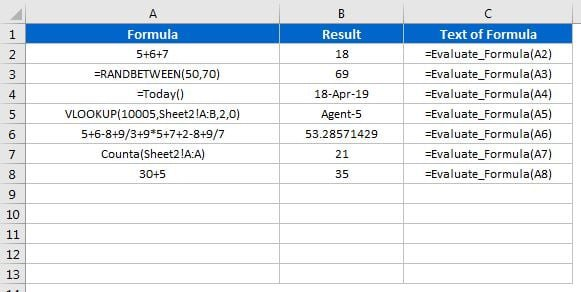Home>Blogs>VBA>UDF for Evaluate a Formula in Excel

# UDF for Evaluate a Formula in Excel

In this article you will learn how to create a User Defined Function to evaluate a formula in Excel. You will also learn how to get text of formula using a User Defined Function and FORMULATEXT (Excel 2013 and above).Below is the code to create the User Defined Function to evaluate a formula in Excel

```Function Evaluate_Formula(formula_text As String) As Variant

Application.Volatile
Evaluate_Formula = Application.Evaluate(VBA.Trim(formula_text))

End Function```

### Text of Formula:

If you are using Excel 2013 and above version, then you can use “=FORMULATEXT” in Excel. For Excel 2010 and below version I have created a User Defined Function.

Below is the code-

```Function Text_Of_Formula(rng As Range) As String

Application.Volatile
Text_Of_Formula = rng.Formula

End Function```

You can copy this code in Personal Macro so that it will be available for each workbook.